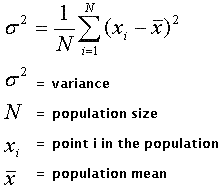Jan 2, 2007 by

What does variance measure?

Variance is a measure of dispersion. Imagine two classrooms, L and H, that contain five students and are given a three question test.

The scores of the students in classroom L on the test are: 2, 2, 2, 2, 2

The scores of the students in classroom H on the test are: 1, 1, 2, 3, 3

Classroom L has low variance, while classroom H has high variance. In both classrooms, the mean (average) score on the test was 2.

One scenario that would produce this outcome is if in classroom L, everyone had been taught the answer to two of the three questions, and everyone remembered all of the answers. Meanwhile, the students in classroom H had been taught the answers to all of the three questions, but remembered them to differing degrees (two students remembered all three, while two students remembered only one).

The formula for variance is:In both classrooms, the population mean is 2 and the population size is 5.

In classroom L, the variance is (1/5)*( (2-2)^2 + (2-2)^2 + (2-2)^2 + (2-2)^2 + (2-2)^2 ) = 0

In classroom H, the variance is (1/5)*( (1-2)^2 + (1-2)^2 + (2-2)^2 + (3-2)^2 + (3-2)^2 ) = 0.8

It should not be a surprise that the variance in classroom H is higher than in classroom L.

Why investors should think about variance

Imagine two investors who had \$12,000 to invest. The investors could invest it in two \$6,000 investments, or in four \$3,000 investments.

• \$6,000 of Stock A
• \$6,000 of Stock B

• \$3,000 of Stock A
• \$3,000 of Stock B
• \$3,000 of Stock C
• \$3,000 of Stock D

By a random twist of fate, stock A and stock C lose half their value, while stock B and stock D gain half their value. Thus, both Investor 1 and Investor 2 end the year with the same amount of money they had at the beginning. However, Investor 1’s portfolio was less diversified, and thus had a higher variance.

Variance of Portfolio 1: (1/2) * ( (3,000 – 6,000)^2 + (9,000 – 6,000)^2 ) = 9,000,000

Variance of Portfolio 2: (1/4) * ( (1,500 – 3,000)^2 + (1,500 – 3,000)^2 + (4,500 – 3,000)^2 + (4,500 – 3,000)^2 ) = 2,250,000

Although Portfolio 1 and Portfolio 2 had the same return, Portfolio 1 had four times the variance of Portfolio 2.

Now, imagine that you could hire either Investor 1 or Investor 2 to be your financial advisor. Which one should you prefer? Investor 2 took far less risk, and got the same return as Investor 1. Imagine that stock A went bankrupt. If that happened, Investor 1 would lose half the value of his portfolio, while Investor 2 would only lose a quarter the value of his portfolio. For a given degree of risk (which corresponds to variance in expected returns) the better investor receives a larger return. Likewise, for a given degree of return, the better investor takes on less risk.

Investing in a larger number of equities reduces the variance of your portfolio. Likewise, a portfolio that has evenly-sized investments has a lower variance than one that has unevenly-sized investments. Take, for example, Investor 3, who invests in three equities like Investor 2, but does so in non-equal quantities.

• \$1,000 of Stock A
• \$1,000 of Stock B
• \$5,000 of Stock C
• \$5,000 of Stock D

As before, A and C lose half their value, while B and D gain half their value.

Variance of Portfolio 3: (1/4) * ( (500 – 1,000)^2 + (1,500 – 1,000)^2 + (2,500 – 5,000)^2 + (7,500 – 5,000)^2 ) = 3,250,000

As you can see, although Investor 3 is investing in four equities like Investor 2, and receiving the same return, he is taking more risk, as his variance is 3,250,000 which is greater than 2,250,000. What is the moral of the story? To reduce the variance of your portfolio diversify (invest in lots of things) and invest evenly in the equities. Doing so will reduce the “risk” you take while investing. You should only invest more in one equity than another if you have substantial evidence that it will perform better than the other-doing so requires you to take a risk, and that risk should be justified.

### Tags

1.2.